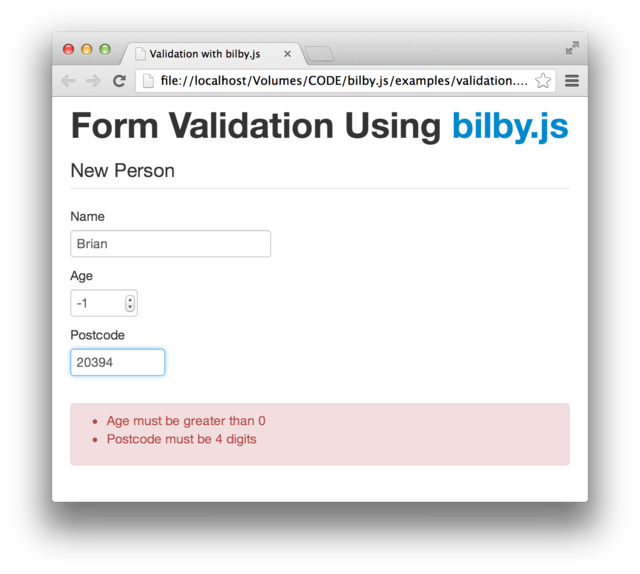BAM Weblog

## Applicative Validation in JavaScript

2013-03-27

Validation is known as scalaz' gateway drug. Validation has the following two states:

• Successful value
• Failure with a Semigroup of errors

Semigroup sounds scary but it's something that can be appended together. Think of a list, array or set. This allows multiple failures to be combined, where the errors are appended together.

People love validation in scalaz because it allows nice, declarative code like the following:

``````case class Person(name: String, age: Int, postcode: String)

postcode <*> (age <*> (name map Person.curried))
``````

What if we could write JavaScript like the following?

``````var Person = _.tagged('Person', ['name', 'age', 'postcode']);

_.Do()(
_.Do()(name < _.curry(Person)) * age * postcode
);
``````

Now you can, with bilby.js:Here is an online demo. Continue reading for details about the implementation.

### Apply

The `<*>` syntax in scalaz is adapted from Haskell's Applicative class. Haskell's class is missing a little abstraction so scalaz introduces a hierarchy like so:

• Functor (map)
• Apply (ap)
• Applicative (pure)

bilby.js contains both Functor and Apply for Validation. Functor means that Validations can be mapped over:

``````_.map(
_.success(1),
function(x) {
return x + 1;
}
);
// Validation.success(2)
``````

A failure value won't be mapped over - it will just be returned. Easy.

Apply means we can run functions from inside of the Validation:

``````_.ap(
_.success(
function(x) {
return x + 1;
}
),
_.success(1)
);
// Validation.success(2)
``````

The above use of `ap` might just look like a different way of writing `map` but look at what happens when there are errors involved:

``````_.ap(
_.failure(['Name must not be empty']),
_.success(1)
);
// Validation.failure(['Name must not be empty'])

_.ap(
_.failure(['Name must not be empty']),
_.failure(['Age must be at least 1'])
);
// Validation.failure(['Name must not be empty', 'Age must be at least 1'])
``````

So all involved errors are accumulated into a single failure.

### Syntax

Sounds good but how do we overload the `<` and `*` operators in JavaScript!?

With a nice hack of a little known JavaScript feature, of course. What happens when we write code like this?

``````{} + {}
``````

JavaScript engines actually lookup a `valueOf` property on each of the objects. It allows things like this:

``````var ten = {valueOf: function() { return 10; }};
var two = {valueOf: function() { return 2; }};
ten + two == 12;
``````

Notice that the `valueOf` is just an arbitrary function that returns a primitive value? We could also use it to perform a side-effect...

When `Do()` is called, a global stack is allocated:

``````doQueue = [];
``````

Then an operator is used in the following expression. For example:

``````_.Do()([1, 2, 3] + [4, 5, 6] + [7, 8, 9]);
``````

On each of the objects, `valueOf` is called and `this` is pushed onto the stack:

``````doQueue.push(this);
``````

So we have a clean stack of all the operands. We can perform a function on that stack and return a value! But how do we know what to return from `valueOf` or which operator was used?

The value returned from `valueOf` can actually tell us which operator was used. In bilby.js, 1 is always returned from the special `valueOf`. We can then use the following logic to figure out the operator:

``````if(n === true) op = 'flatMap'; // >=
if(n === false) op = 'map'; // <
if(n === 0) op = 'kleisli'; // >>
if(n === 1) op = 'ap'; // *
if(n === doQueue.length) op = 'append'; // +
``````

Stepping through the above example:

``````Do() // doQueue = []

[1, 2, 3].valueOf() // doQueue.push([1, 2, 3]), 1
[4, 5, 6].valueOf() // doQueue.push([4, 5, 6]), 1
[7, 8, 9].valueOf() // doQueue.push([7, 8, 9]), 1
1 + 1 + 1 // 3

// 3 is doQueue.length; '+' must be the operator.

return [1, 2, 3].append([4, 5, 6]).append([7, 8, 9]);
``````

Almost magical...

Now put it all together and the following plain JavaScript:

``````_.Do()(
_.Do()(name < _.curry(Person)) * age * postcode
);
``````

Desugars to this:

``````_.ap(_.ap(_.map(name, f), age), postcode);
``````

### Summary

We can apply category theory to derive a readable, declarative validation API in JavaScript. The theory is based around Functors, Applicatives and Semigroups. A total of about 3 abstract functions.

We can (ab)use JavaScript's magical `valueOf` property to achieve operator overloading and provide an even more declarative syntax.

If you'd like to like to find out more about validation in bilby.js, please read the documentation. If you want to find out more about the implementation, head to bilby.js' GitHub repository.